# An Extensive Guide for Health Care Data Analysis using R(Machine Learning Algorithms, GLM)

1. Data exploration (Data distribution inferences, Univariate Data analysis, Two-sample t-test)

2. Data Correlation Analysis

3. Feature Selection (using Logistic regression)

4. Outlier Detection (using principal component graph)

5. Basic Parameter Tuning(CV, complexity parameter)

6. Data modeling

Basic GLM (With all Features and eliminating few features based on AIC)

Logistic Regression

Decision Tree

Naïve BayesBasic EDA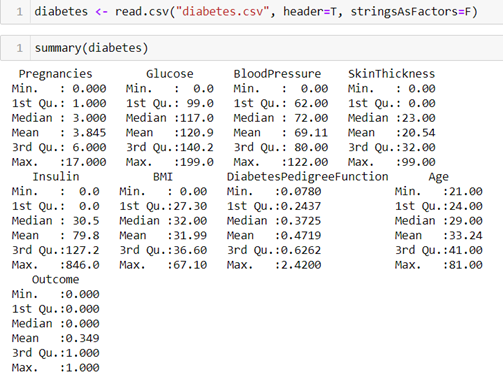# Univariate analysis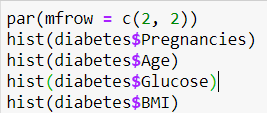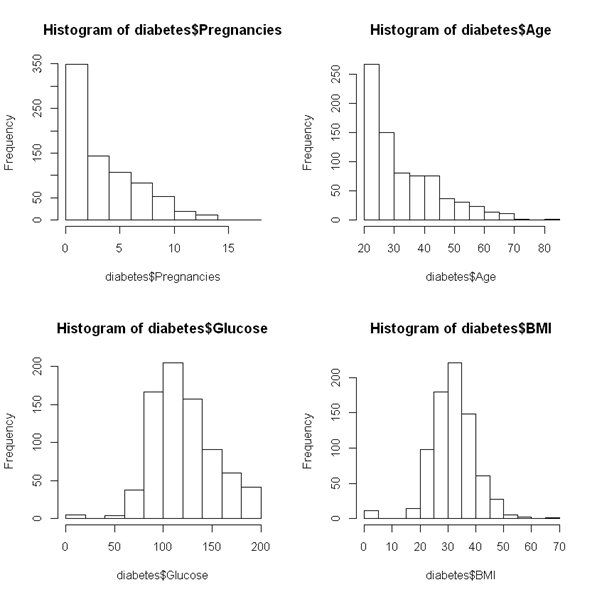From these distribution graphs,

Age and number of times pregnant are not in normal distributions as expected since the underlying population should not be normally distributed either.

Glucose level and BMI are following a normal distribution.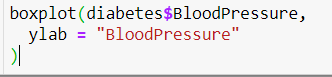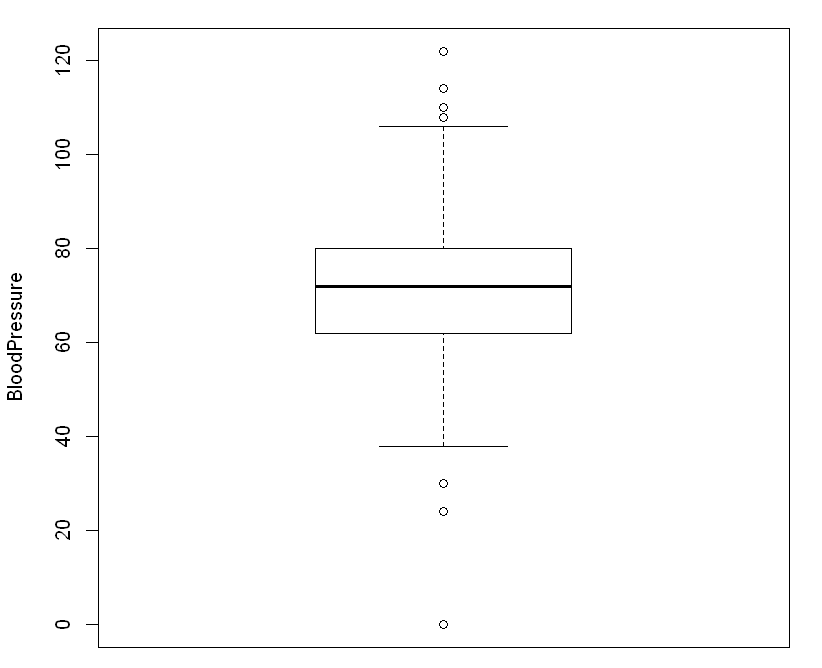# Impact of Glucose on Diabetes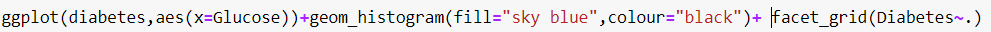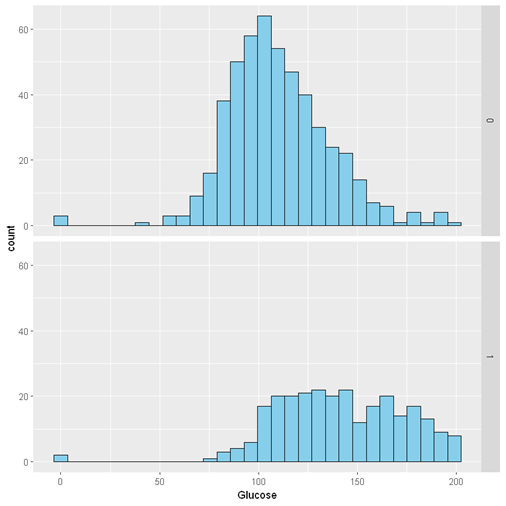Formulate a hypothesis to assess the mean difference of glucose levels between the positive and negative groups.

Conditions

Individuals are independent of each other

Here distributions are skewed but the sample >30

Both the groups are independent of each other and the sample size is lesser than 10% of the population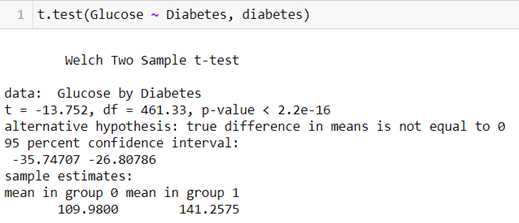p-value is < critical values of 0.05, so we reject the null hypothesis for the alternate hypothesis. We can say that we are, 95 % confident, that the average glucose levels for individuals with diabetes is > the people without diabetes.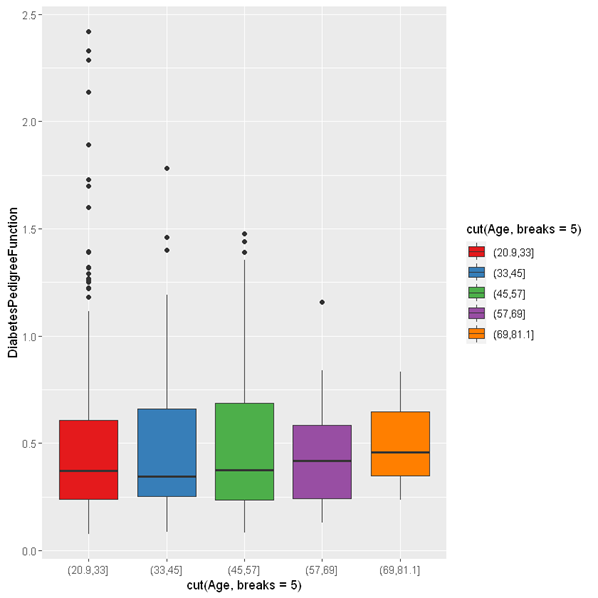Insulin Vs Glucose based on Outcome as diabetes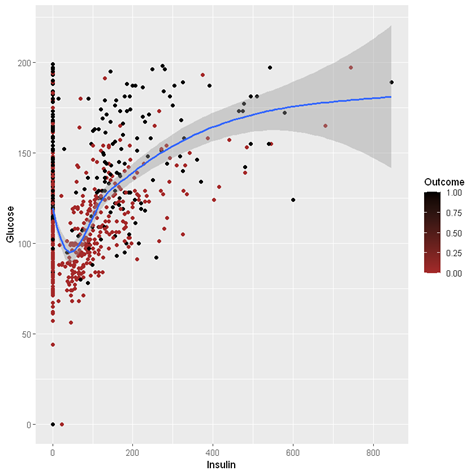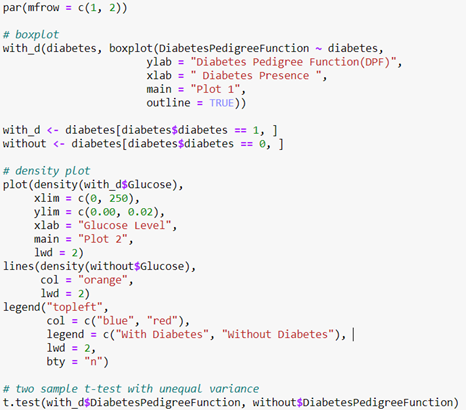Welch Two Sample t-test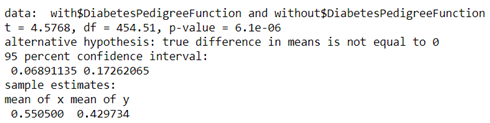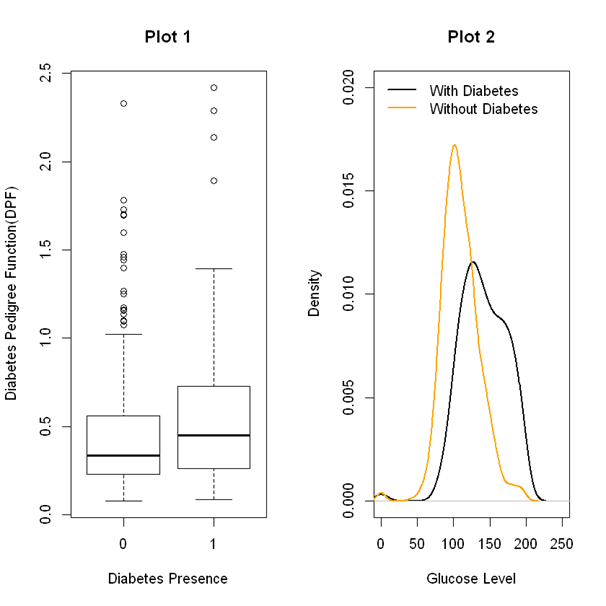From Plot 2 the distribution is shifted towards the left for those without diabetes.

This indicates those without diabetes generally have a lower blood glucose level.

Correlation between each variable

Scatter matrix of all columns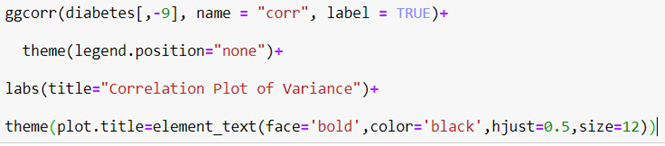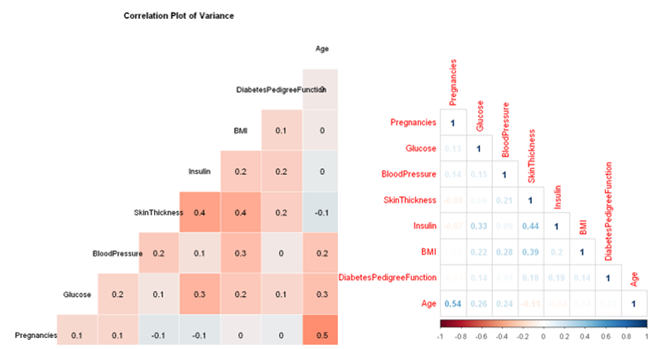Pregnancy, Age, Insulin, skinthickness are having higher correlation.

Fitting a logistic regression to assess importance of predictors

• Fitting a GLM (General Linear Model) with link function ‘probit’

• Target variable ‘diabetes’ estimated to be binomially distributed

This is a generic implementation — without assumption on data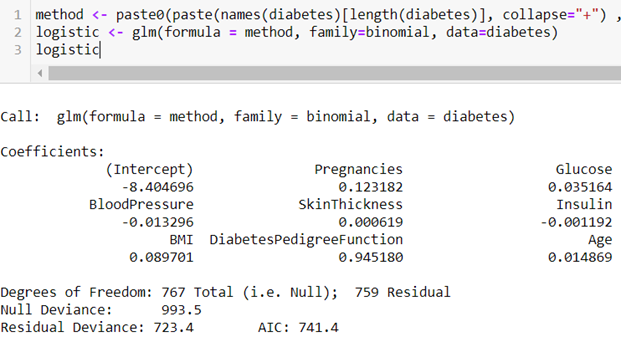# Filtering the most important predictors from GLM model

• Extracting the N most important GLM coefficients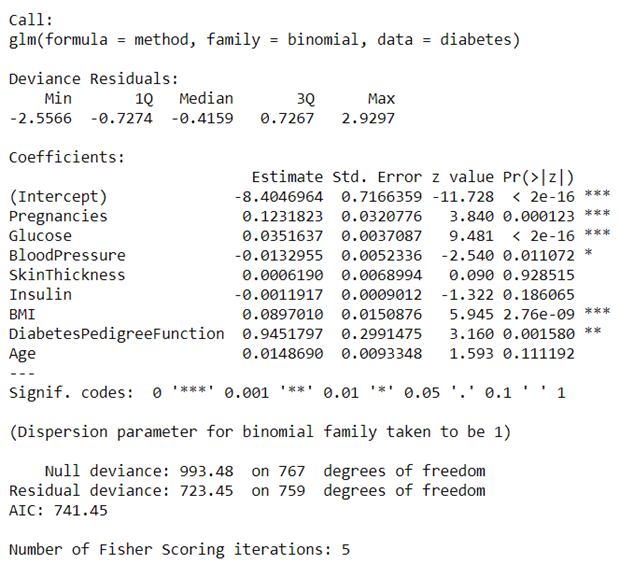• Logistic Regression for: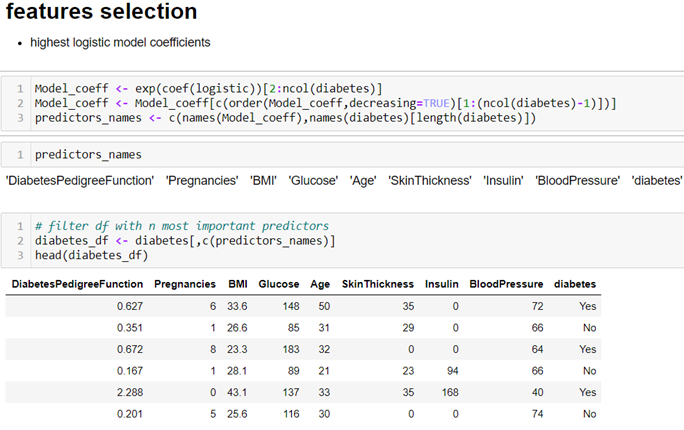Outlier Detection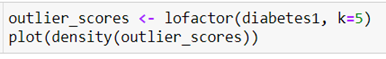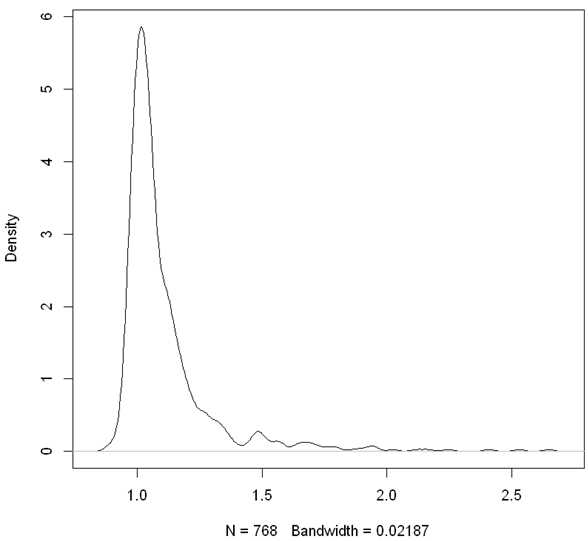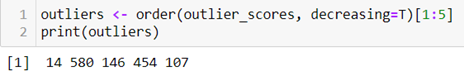# The five outliers obtained in the output are the row numbers in the diabetes1 data derived from the diabetes data set.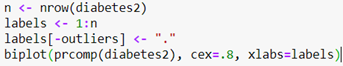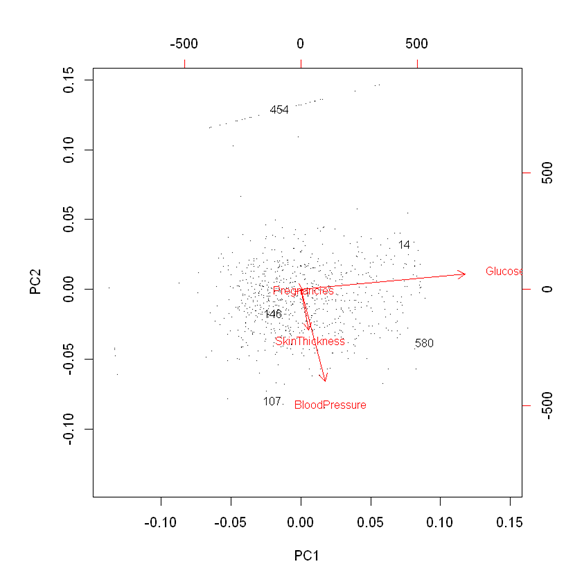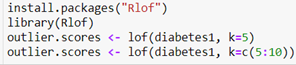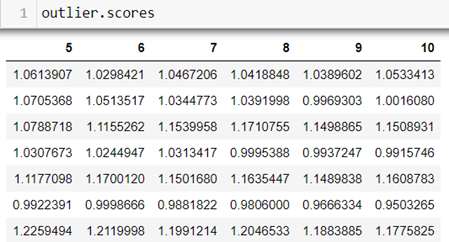Data Modelling

1. Basic GLM with all Variables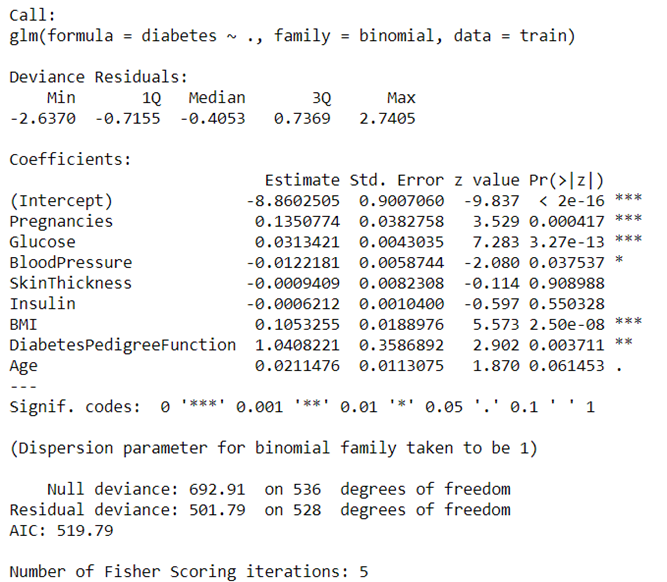The result shows that the variables Triceps_Skin, Serum_Insulin and Age are not statistically significant. p_values is > 0.01 so we can experiment by removing it.

# Logistic Model

input: explanatory variables xk and provides a prediction p with parameters βk.

The logit transformation constrains the value of p to the interval [0, 1].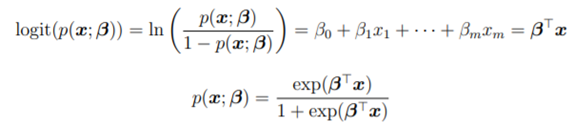βk represents the log-odds of feature xk says how much the logarithm of the odds of a positive outcome (i.e. the logit transform) increases when predictor xk increases by .

Likelihood of the model as follows: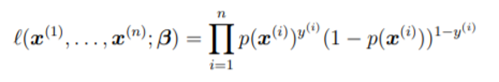Y^i = outcome of subject i.

Maximizing the likelihood = maximizing the log-likelihood(model)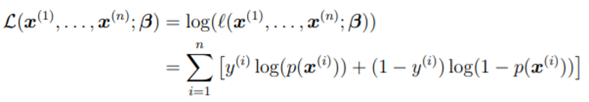The above equation is non-linear for logistic regression and its minimization is generally done numerically by iteratively re-weighted least-squares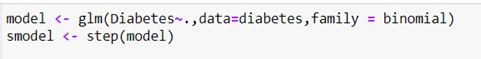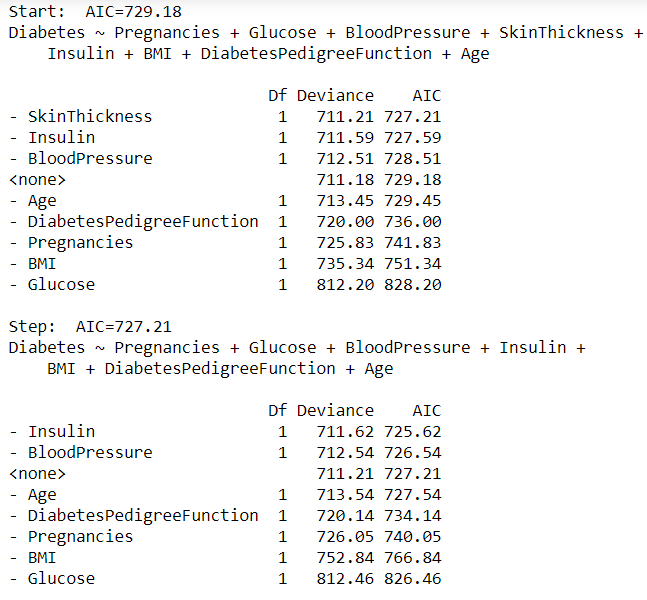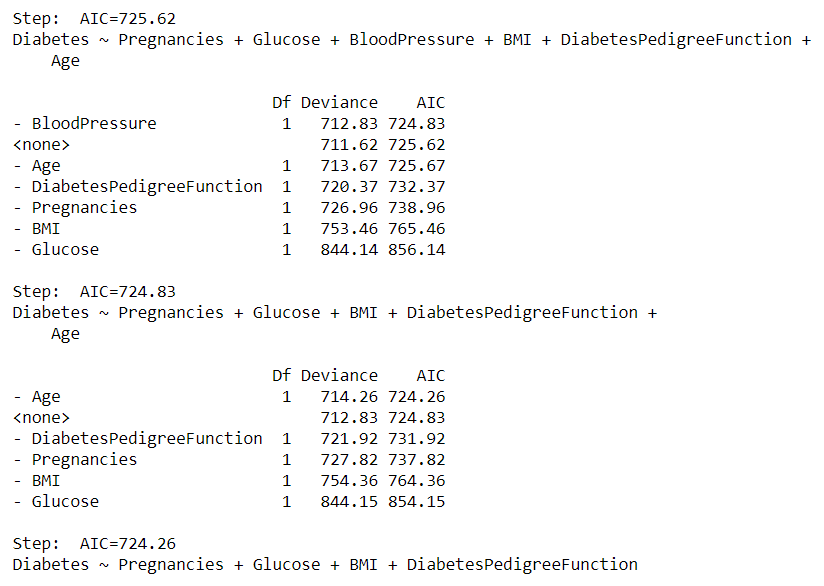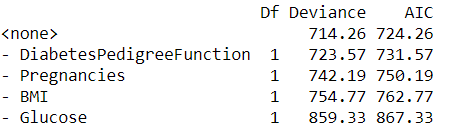# Initial Parameter Tuning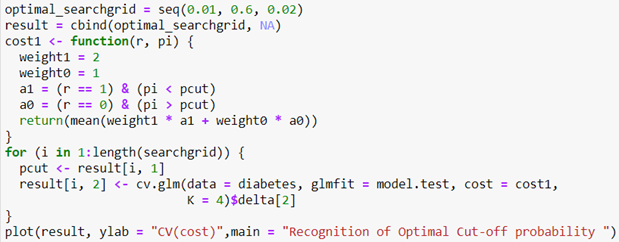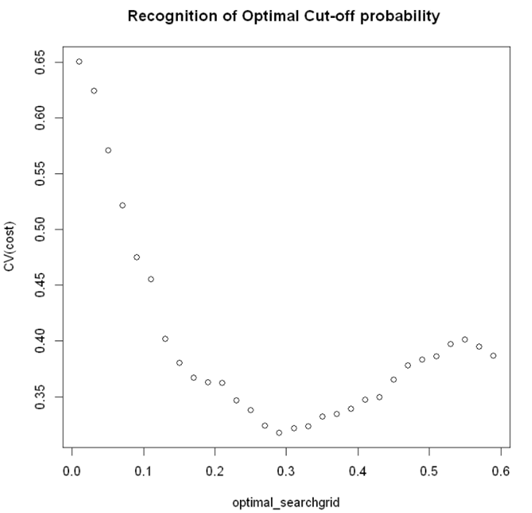From this graph, the cross-validated cost pcut 0.28 is chosen as the optimal cut-off probability with a CV cost of 0.3370.

tree <- rpart(Diabetes~., data=diabetes, method=”class”)

rpart.plot(tree)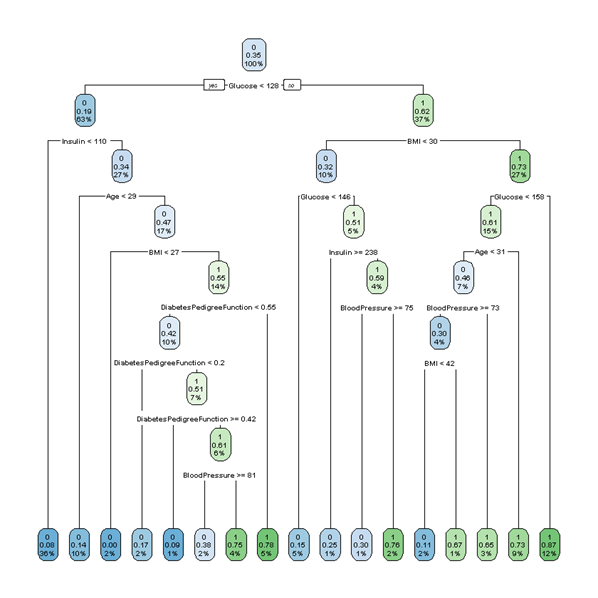plotcp(tree)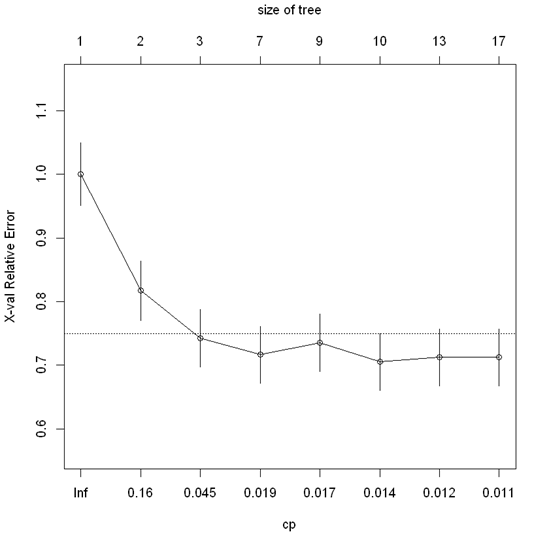Complexity parameter

The above tree was tuned using a reference of the Relative error VS Complexity parameter. From the above figure the Cp value of 0.016, the decision tree was pruned. The final decision tree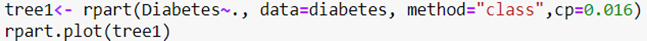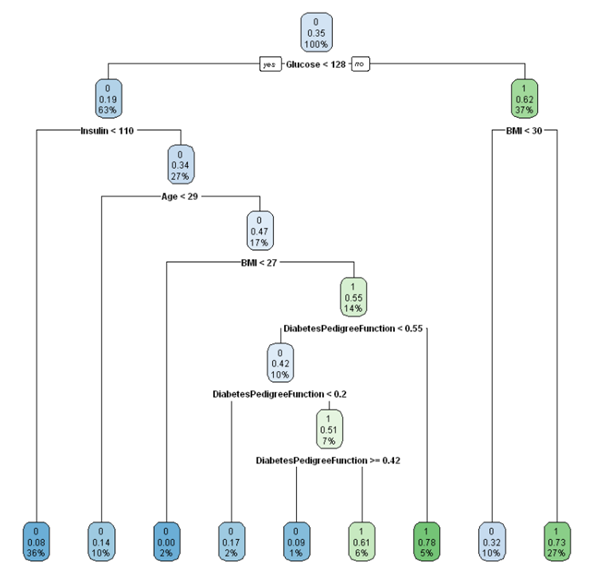If CP value is lower, tree will grow large. A cp = 1 will provide no tree which helps in pruning the tree. Higher complexity parameters can lead to an over pruned tree.

2nd Model By removing 3 features-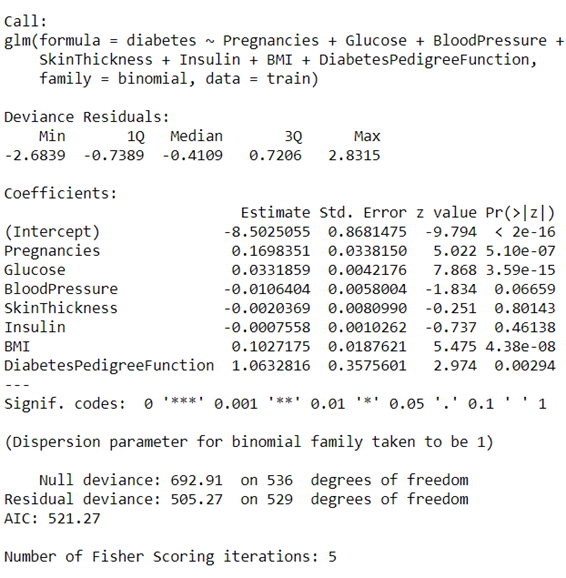par(mfrow = c(2,2))

plot(glm_m2)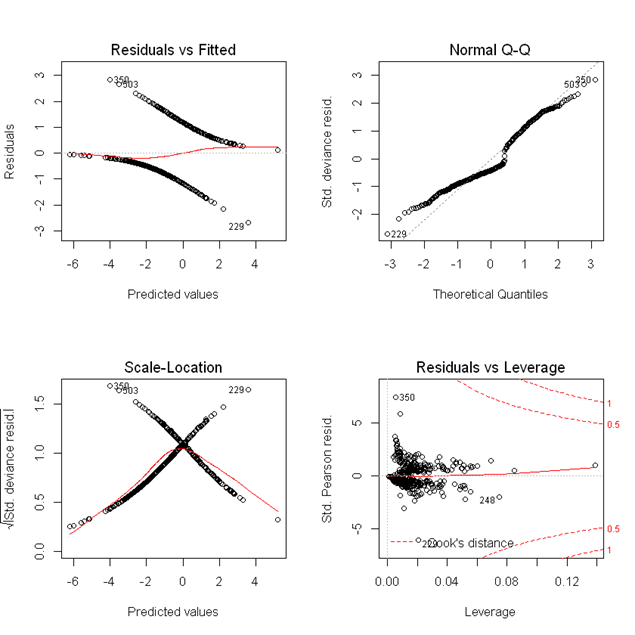1. Residuals vs fitted values; Here dotted line at y=0 indicates fit line. The points on fit line indication of zero residual. Points above are having positive residuals similarly points below have negative residuals. . The red line is indicates smoothed high order polynomial curve which provides the idea behind pattern of residual movements. Here the residuals have logarithmic pattern hence we got a good model.

2. Normal Q-Q Plot: In general Normal Q-Q plot is used to check if our residuals follow Normal distribution or not. The residuals are said to be normally distributed if points follow the dotted line closely.

In our case residual points follow the dotted line closely except for observation at 229, 350 and 503 So this model residuals passed the test of Normality.

3. Scale — Location Plot: It indicates spread of points across predicted values range.

Assumption:

- Variance should be reasonably equal across the predictor range(Homoscedasticity)

So this horizontal red line is set to be ideal and it indicates that residuals have uniform variance across the Predictor range. As residuals spread wider from each other the red spread line goes up. In this case the data is Homoscedastic i.e having uniform variance.

4. Residuals vs Leverage Plot:

Influence: The Influence of an observation can be defined in terms of how much the predicted scores would change if the observation is excluded. Cook’s Distance

Leverage: The leverage of an observation is defined on how much the observation’s value on the predictor variable differs from the mean of the predictor variable. The more the leverage of an observation , the greater potential that point has in terms of influence.

In our plot the dotted red lines are the cook’s distance and the areas of interest for us are the ones outside the dotted line on the top right corner or bottom right corner. If any point falls in that region, we say the observation has high leverage or having some potential for influencing our model is higher if we exclude that point.

3rd Model: Predict Diabetes Risk on new patients using Decision Tree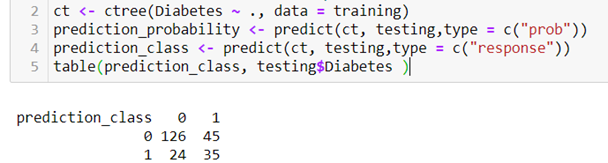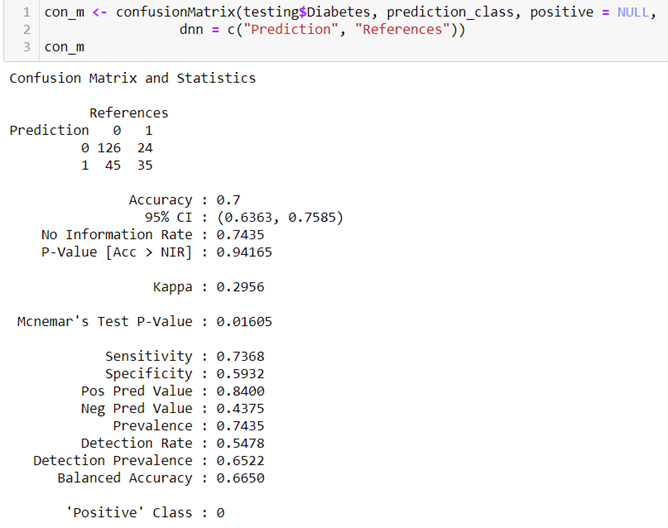4th Model Naïve Bayes: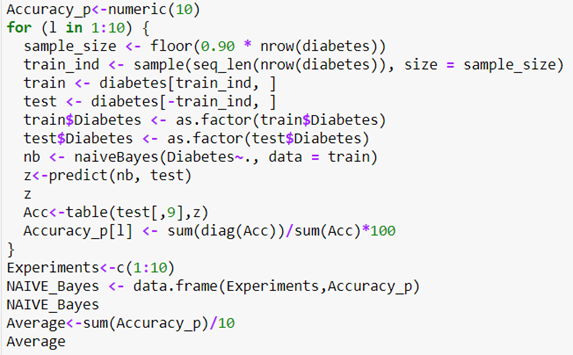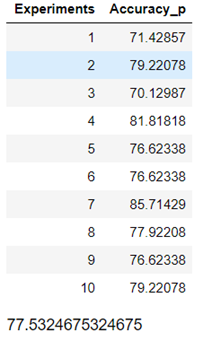Though it’s a basic model still it performed well with 77% accuracy on an average

References:

https://online.stat.psu.edu/stat508/lesson/3/3.5